Download PresentationChaos

# Chaos - PowerPoint PPT Presentation

Chaos Chow Yuen Shan Chu Wai Siu Outline Introduction Brief history of the discovery of chaos Working definition of chaos Chaos in simple deterministic systems Logistic map Higher dimensional models Examples outside Physics Implications History 1890s Poincaré : three – body problemI am the owner, or an agent authorized to act on behalf of the owner, of the copyrighted work described.
Download Presentation## Chaos

An Image/Link below is provided (as is) to download presentation

Download Policy: Content on the Website is provided to you AS IS for your information and personal use and may not be sold / licensed / shared on other websites without getting consent from its author.While downloading, if for some reason you are not able to download a presentation, the publisher may have deleted the file from their server.

- - - - - - - - - - - - - - - - - - - - - - - - - - E N D - - - - - - - - - - - - - - - - - - - - - - - - - -
Presentation Transcript### Chaos

Chow Yuen Shan

Chu Wai Siu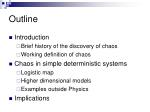Outline

Introduction

Brief history of the discovery of chaos

Working definition of chaos

Chaos in simple deterministic systems

Logistic map

Higher dimensional models

Examples outside Physics

Implications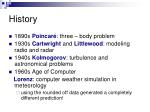History

1890s Poincaré: three – body problem

1930s Cartwrightand Littlewood: modeling radio and radar

1940s Kolmogorov: turbulence and astronomical problems

1960s Age of Computer

Lorenz: computer weather simulation in meteorology

using the rounded off data generated a completely different prediction!History

1960s Mandelbrot: Recurring patterns at every scale (Self – similarity), “measuring the coast of Britain (Fractional dimensions)

1970s Robert May: Logistic map in biology

Simplest deterministic model showing chaotic behavior

Mandelbrot Set

Graph from http://en.wikipedia.org/wiki/Mandelbrot_set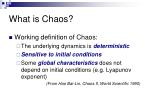What is Chaos?

Working definition of Chaos:

The underlying dynamics is deterministic

Sensitive to initial conditions

Some global characteristicsdoes not depend on initial conditions (e.g. Lyapunov exponent)

(From Hao Bai-Lin, Chaos II, World Scientific 1990)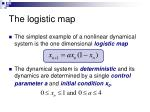The logistic map

The simplest example of a nonlinear dynamical system is the one dimensional logistic map

The dynamical system is deterministicand its dynamics are determined by a single control parameter aandinitial condition x0.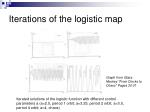Iterations of the logistic map

Graph from Glass Mackey “From Clocks to Chaos”Pages 30-31

Iterated solutions of the logistic function with different control parameters a (a=2.5, period 1 orbit; a=3.25, period 2 orbit; a=3.5, period 4 orbit; a=4, chaos)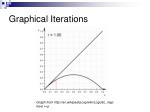Graphical Iterations

Graph from http://en.wikipedia.org/wiki/Logistic_map here r=a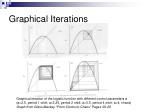Graphical Iterations

Graphical iteration of the logistic function with different control parameters a (a=2.5, period 1 orbit; a=3.25, period 2 orbit; a=3.5, period 4 orbit; a=4, chaos)

Graph from Glass Mackey “From Clocks to Chaos” Pages 28-29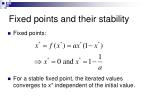Fixed points and their stability

Fixed points:

For a stable fixed point, the iterated values converges to x* independent of the initial value.Stability of Fixed Points
• Super-stable fixed point, which converges very fast
• To test the stability of the 2 fixed points:Multiple Fixed Points

It is possible that the trajectory of iteration has more than one fixed point:

The stability is given by:

Where x1 and x2 are the two fixed pointsPeriod-doubling

For a > 3 , fixed point becomes unstable and bifurcates to a cycle of period 2.

As a increases, the fixed points of f(2)(x) become unstable and cycle period 4 appears, and so forth.

A sequence of period-doublings accumulating to a chaotic trajectory of infinite period atBifurcation diagram

Bifurcation diagram for the logistic function: post-transient solution against control parameter. (http://mathworld.wolfram.com/LogisticMap.html)In the chaotic regime
• The evolution of the difference Δxn between the trajectories at a=3.64 for x0 = 0.5 and x0 = 0.5001
• The separation increases with n

Graph from Harvey Gould “An Introduction to Computer Simulation Methods” Page 147

Sensitive to initial conditions

Even the system is deterministic, the ability to make long – term predictions is limited.Some Universal Properties

Some numerical evidence shows that the general behavior of the logistic map is independent of the details form of the function

The range of a between successive bifurcations becomes smaller as the period increases.

The ratio converges to a constant with increasing k.Some Universal Properties

Values of ak for the onset of the kth bifurcation

Graph from Harvey Gould “An Introduction to Computer Simulation Methods”Some Universal Properties
• The Feigenbaum number is defined to be:
• The value was found to be:Some Universal Properties
• We can also consider the distance
• is the value of the fixed point nearest to the fixed point

Graph from Harvey Gould “An Introduction to Computer Simulation Methods”Some Universal Properties
• We define
• The value of αwas found to be
• Feigenbaum showed that the values of δ and α are universal property of maps that have a quadratic maximum, i.e.Self-similarity

Graph from http://www.calresco.org/beckermn/nonlindy.htm

The period-doubling look similar except for a change of scale.(a) f(x) for a=2 and (b) f(2)(x) for a = 3.236.

Graph from Harvey Gould “An Introduction to Computer Simulation Methods” Page 145

If the square in (b) is scaled up δ horizontally and α vertically, and flipped about x=0.5, it will nearly cover that in (a)

The error decreases when a increases.Measuring Chaos

The evolution of the difference Δxn between the trajectories at a=3.64 for x0 = 0.5 and x0 = 0.5001

Graph from Harvey Gould “An Introduction to Computer Simulation Methods” Page 147

How do we know if a system is chaotic?

By measuring the its sensitivity to initial conditions.Average Lyapunov Exponent

Consider x1 and x2=x1+δx1, with N time steps long, thenAverage Lyapunov Exponent

Assume

The system is chaotic ifAverage Lyapunov Exponent as a function of r = a/4

Graph from Harvey Gould “An Introduction to Computer Simulation Methods” Page 149Quick Summary:
• Simple deterministic models can generate chaos
• Characteristics of chaotic systems:
• Period-doubling is one of the most understandable routes to chaos
• Sensitivity to initial conditions
• Universal constants can be defined
• Exhibits self-similarity
• Positive Lyapunov exponentConcepts of attractor
• A dynamical system consists of two parts:
• the notions of a state (the essential information about the system )
• a dynamic ( a rule that describes how the state evolves with time )
• The evolution can be visualized in a state space, an abstract construct whose coordinates are the degrees of freedom of the system’s motion.
• An attractor is what the behavior of a system settles down to.Example: simple pendulum
• The state is completely specified by the position and velocity.
• The rule is Newton’s law.
• As the pendulum swings, the state moves along an orbit in the state space

Graph from J P Crutchfield et al, “Chaos”, Sci Amer 254 45 (1987)• Examples are pendulum subject to friction, stable oscillations such as heart beat, compound oscillations respectivly.
• They are predictable motion.

Graph from J P Crutchfield et al, “Chaos”, Sci Amer 254 45 (1987) showing the 3 types of attractors

Graph from Glass Mackey “From Clocks to Chaos”The Lorenz Model (1963)
• x is the fluid flow velocity
• y is the temperature difference between the rising and falling fluid regions
• z is the difference in temperature between the top and the bottom from the equilibrium state
• The parameters σ, r and b are determined by the fluid properties, size of the system and the initial temperature difference

Used as an atmospheric model

Describe the motion of a fluid layer that is heated from belowLorenz found out that his model could not be characterized by any of the three attractors known.
• The attractor he observed, now known as the Lorenz attractor, was the first example of a chaotic or strange attractor.

Picture from http://en.wikipedia.org/wiki/Lorenz_attractor and complex.upf.es/~josep/Chaos.htmlPicture from www.reinhardkargl.com and Harvey Gould “An Introduction to Computer Simulation Methods” Page 156. Trajectories of Lorenz model with σ = 10, r = 28 and b = 8/3 with initial conditions x=1, y=1 and z=20The Butterfly Effect

Graph from J P Crutchfield et al, “Chaos”, Sci Amer 254 45 (1987) showing the divergence of nearby trajectories.

Nearby trajectories diverge

Final state can be anywhere on the attractor

Long-term prediction is impossibleShort note on Fractal Dimension

Picture from http://en.wikipedia.org/wiki/Fractal_dimension

Strange attractors have non-integer dimension

The most common definition is the Hausdorff dimension.

Divide an object into N identical pieces of length l,then N=1/lDShort note on Fractal Dimension

Picture from http://en.wikipedia.org/wiki/Fractal_dimension

The fractal dimension, D, indicates of how completely a fractal appears to fill space, as one zooms down to finer scales.

Example: Sierpinski triangleShort note on Fractal Dimension

To find the fractal dimension of dynamical systems, divide the space into boxes with length l, N(l) will be the number of boxes that contain part of the attractor

Lorenz attractor: D=2.06±0.01(Grassberger and Procaccia 1983)

Logistic map: D=0.538 (Grassberger 1981)Another example

Picture from http://en.wikipedia.org/wiki/H%C3%A9non_map showing the bifurcation diagram and the attractor with a=1.4 and b=0.3

Hénon map

To study behavior of asteroids and satellitesExamples: Physical systems

Picture from'Regular and Chaotic Behaviour in an Extensible Pendulum'. R. Carretero-González, H.N. Núñez-Yépez and A.L. Salas-Brito. Eur. J. Phys. 15, 3 (1994) 139-148 showing the system and the chaotic trajectory

Picture from http://en.wikipedia.org/wiki/Double_pendulum showing the system and the trajectory

Spring pendulum:

Double pendulum:Beyond Physics

Chaos is observed in every aspects of life:

Economy: Economic bubbles

Weather forecast: El Nino phenomenon

Sociology: Strange Attractor dynamics for the popularity of a corrupt politician (Rinaldi et al, 1994)

Biology: Heart beat, Neural networks

Chemical reactions

Ocean dynamics

Ecology: population of animal speciesImplications
• In the past, scientist believe that the laws of nature imply strict determinism and complete predictability, only imperfections in observations make the introduction of probabilistic theory necessary.

Given the position and velocity of every particle in the universe, I could predict the future for the rest of time.

Picture from http://en.wikipedia.org/wiki/Laplace showing Laplace (1749-1827)Implications – downfall of determinism
• 20th century science has been the downfall of Laplacian determinism, for two reasons:
• Heisenberg uncertainty principle
• Exponential amplification of errors due to chaotic dynamics
• Quantum mechanics implies that initial measurements are always uncertain, and chaos ensures that the uncertainties grow and quickly overwhelm the ability to make predictions.Implications – affects scientific method
• The discovery of chaos has created a new paradigm in scientific modeling:
• new fundamental limits on the ability to make predictions
• many phenomena are more predictable than had been thought
• Affects the scientific method:
• The process of verifying a theory becomes a much more delicate operation.Implications - new questions are raised
• Will chaos persists in microscopic physical systems where the theory of quantum mechanics is expected to apply?
• Quantum mechanics is presumed to be the fundamental theory for all physical systems
• Predictions of quantum theory must agree with those of classical mechanics at the limit of the highest quantum numbers
• But the Schrodinger equation is a linear equation which is incapable of exhibiting the chaotic behavior of nonlinear classical systems!References
• Publications:
• R V Jensen “Classical chaos” Am Scientist 75 168 (1987)
• J P Crutchfield et al, “Chaos”, Sci Amer 254 45 (1987)
• R. Carretero-González, H.N. Núñez-Yépez and A.L. Salas-Brito,“Regular and Chaotic Behaviour in an Extensible Pendulum”. Eur. J. Phys. 15, 3 (1994)
• H M Lai, “On the recurrence phenomenon of a resonant spring pendulum”, Am J Phys 52, 219 (1984)
• Ary L. Goldberger (2000)Nonlinear Dynamics, Fractals, and Chaos Theory: Implications for Neuroautonomic Heart Rate Control in Health and Disease, PhysioNet
• Smith, R. D. (1998) 'Social Structures and Chaos Theory‘ Sociological Research Online, vol. 3, no. 1References
• Books:
• Glass Mackey “From Clocks to Chaos”, (Princeton U Press, 1988)
• J Frφyland “Introduction to chaos and coherence”
• Harvey Gould “An Introduction to Computer Simulation Methods”, (Addison-Wesley, 1996)
• Hao Bai-Lin, “Chaos II”, (World Scientific 1990)
• Websites:
• http://www.calresco.org/beckermn/nonlindy.htm
• http://www.reinhardkargl.com
• http://en.wikipedia.org/wiki/ (topics include: Logistic map, Chaos theory, Attractor, Fractal dimension)
• http://mathworld.wolfram.com/ (topics include: Logistic map, Attractor, Lorenz attractor, Strange attractor, Fractal dimension)